1. 单变量隐函数

F(x,y)=0

[存在定理]  设函数F(x,y)在点M0(x0,y0)的某一邻域*R内定义并且满足下列条件:

(i) F(x,y)及其偏导数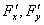R内连续,

(ii) F(x0,y0)=0,

(iii)0,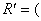;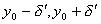)

F[x, f (x)]0,f (x0)=y0,

在区间(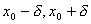)内函数f(x)连续,

它在这区间内有连续的导数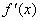.

[导数的计算]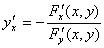(0)(0)

* 邻域的概念见第二十一章，这里M0的领域是指包含M0的某一矩形